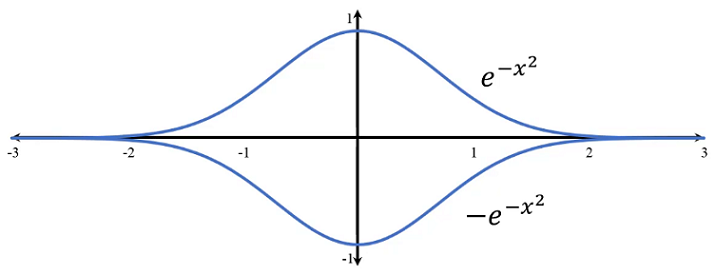# Circle Inscribed in Bell Curves

Calculus Level 3Find the area of the circle that is centered at the origin $(0,0)$ and inscribed between the bell curves with equations: \begin{aligned} y&=e^{-x^2}\\ y&=-e^{-x^2} \end{aligned}

×

Problem Loading...

Note Loading...

Set Loading...